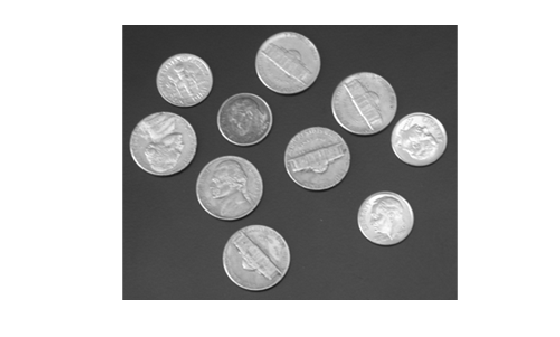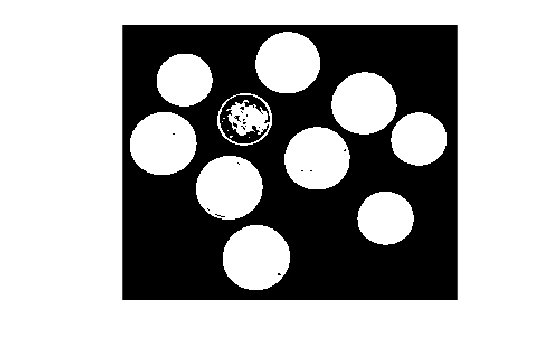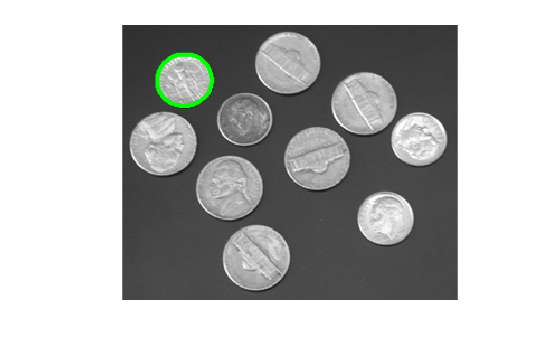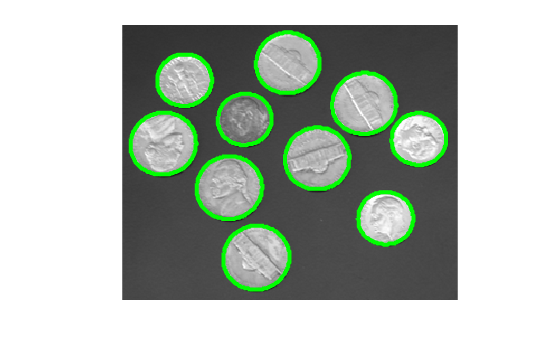## Boundary Tracing in Images

The toolbox includes two functions you can use to find the boundaries of objects in a binary image:

The `bwtraceboundary` function returns the row and column coordinates of all the pixels on the border of an object in an image. You must specify the location of a border pixel on the object as the starting point for the trace.

The `bwboundaries` function returns the row and column coordinates of border pixels of all the objects in an image.

For both functions, the nonzero pixels in the binary image belong to an object, and pixels with the value 0 (zero) constitute the background.

### Trace Boundaries of Objects in Images

This example shows how to trace the border of an object in a binary image using `bwtraceboundary` . Then, using `bwboundaries` , the example traces the borders of all the objects in the image.

Read image and display it.

```I = imread('coins.png'); imshow(I)```Convert the image to a binary image. `bwtraceboundary` and `bwboundaries` only work with binary images.

```BW = im2bw(I); imshow(BW)```Determine the row and column coordinates of a pixel on the border of the object you want to trace. `bwboundary` uses this point as the starting location for the boundary tracing.

`dim = size(BW)`
```dim = 1×2 246 300 ```
```col = round(dim(2)/2)-90; row = min(find(BW(:,col)))```
```row = 27 ```

Call `bwtraceboundary` to trace the boundary from the specified point. As required arguments, you must specify a binary image, the row and column coordinates of the starting point, and the direction of the first step. The example specifies north ( `'N'` ).

`boundary = bwtraceboundary(BW,[row, col],'N');`

Display the original grayscale image and use the coordinates returned by `bwtraceboundary` to plot the border on the image.

```imshow(I) hold on; plot(boundary(:,2),boundary(:,1),'g','LineWidth',3);```To trace the boundaries of all the coins in the image, use the `bwboundaries` function. By default, `bwboundaries` finds the boundaries of all objects in an image, including objects inside other objects. In the binary image used in this example, some of the coins contain black areas that `bwboundaries` interprets as separate objects. To ensure that `bwboundaries` only traces the coins, use `imfill` to fill the area inside each coin. `bwboundaries` returns a cell array, where each cell contains the row/column coordinates for an object in the image.

```BW_filled = imfill(BW,'holes'); boundaries = bwboundaries(BW_filled);```

Plot the borders of all the coins on the original grayscale image using the coordinates returned by `bwboundaries` .

```for k=1:10 b = boundaries{k}; plot(b(:,2),b(:,1),'g','LineWidth',3); end```### Select First Step and Direction for Tracing

For certain objects, you must take care when selecting the border pixel you choose as the starting point and the direction you choose for the first step parameter (north, south, etc.).

For example, if an object contains a hole and you select a pixel on a thin part of the object as the starting pixel, you can trace the outside border of the object or the inside border of the hole, depending on the direction you choose for the first step. For filled objects, the direction you select for the first step parameter is not as important.

To illustrate, this figure shows the pixels traced when the starting pixel is on a thin part of the object and the first step is set to north and south. The connectivity is set to 8 (the default).

Impact of First Step and Direction Parameters on Boundary Tracing